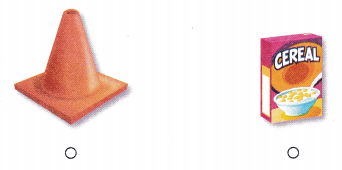# Texas Go Math Kindergarten Lesson 18.2 Answer Key Identify Cones

Refer to our Texas Go Math Kindergarten Answer Key Pdf to score good marks in the exams. Test yourself by practicing the problems from Texas Go Math Kindergarten Lesson 18.2 Answer Key Identify Cones.

## Texas Go Math Kindergarten Lesson 18.2 Answer Key Identify Cones

Essential Question
How do you sort, classify, and Identify cones?

ExploreExplanation:
I drew shapes that are cones and that are not cones.

Directions
Use three-dimensional shapes and identify the cone. Sort the shapes. Describe the cone. Match a picture of each shape to the shapes on the sorting mat. Glue the shape pictures to show how you sorted.

Share and Show

Question 1.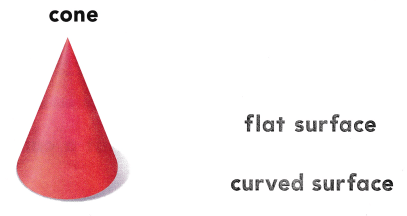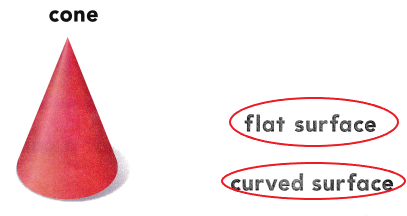Explanation:
A cone has a flat surface and a curved surface
So, i drew circles around the words that describe a cone.

Question 2.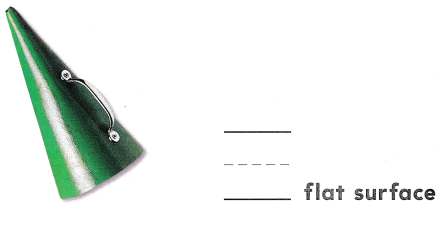Explanation:
A cone has 1 flat surface. So, i wrote the number 1.

Directions
1. Look at the cone. Circle the words that describe a cone. 2. Use a cone to count how many flat surfaces. Write the number.

Question 3.Explanation:
I identified the cones shaped objects and marked X on those objects.

Directions
3. Identify the objects that are shaped like a cone. Mark an X on those objects.

Home Activity

• Have your child identify and describe a household object that is shaped like a cone.

Problem Solving

Question 4.Explanation:
I marked X on the shape that has a flat surface and a curved surface.

Question 5.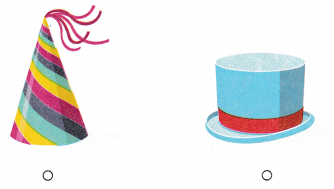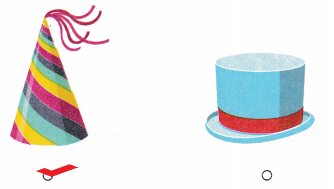Explanation:
I checked the hat that is shaped like a cone.

Directions
4. I have a curved surface and 1 flat surface. Which shape am I? Mark on X on that shape 5. Choose the correct answer. Which hat is shaped like a cone?

### Texas Go Math Kindergarten Lesson 18.2 Homework and Practice

Question 1.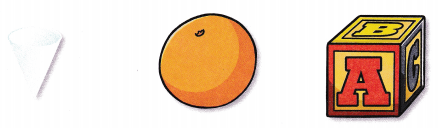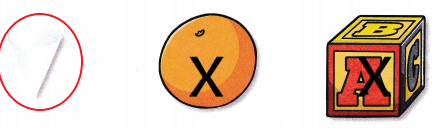Explanation:
I drew a circle around the object that is shaped i like a cone and marked X on the objects that are not shaped like a cone.

Question 2.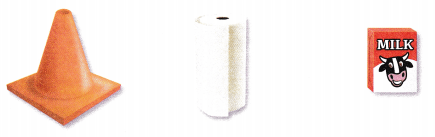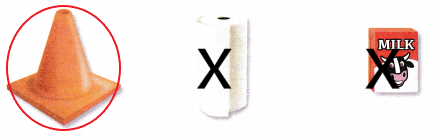Explanation:
I drew a circle around the object that is shaped i like a cone and marked X on the objects that are not shaped like a cone.

Question 3.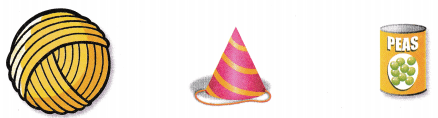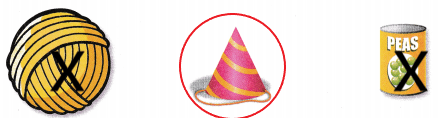Explanation:
I drew a circle around the object that is shaped i like a cone and marked X on the objects that are not shaped like a cone.

Directions
1. Sort the shapes. Circle the object shaped like a cone. Mark on X on the objects that are not shaped like a cone. 2-3. Sort the shapes. Circle the object that is shaped like a cone. Mark an X on the objects that are not shaped like a cone.

Texas Test Prep

Lesson Check

Question 4.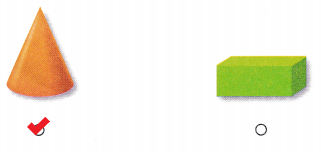Explanation:
I checked the block that is in the shape of a cone.

Question 5.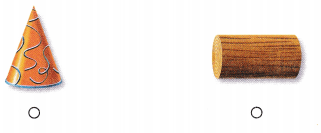Explanation:
I checked the object that is not a cone.

Question 6.